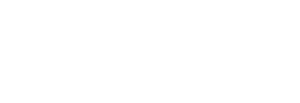# Start preparing for AMC 10 Geometry

There are two components of the geometry course for AMC 10: the concepts and the problems.

# Graph of Knowledge

•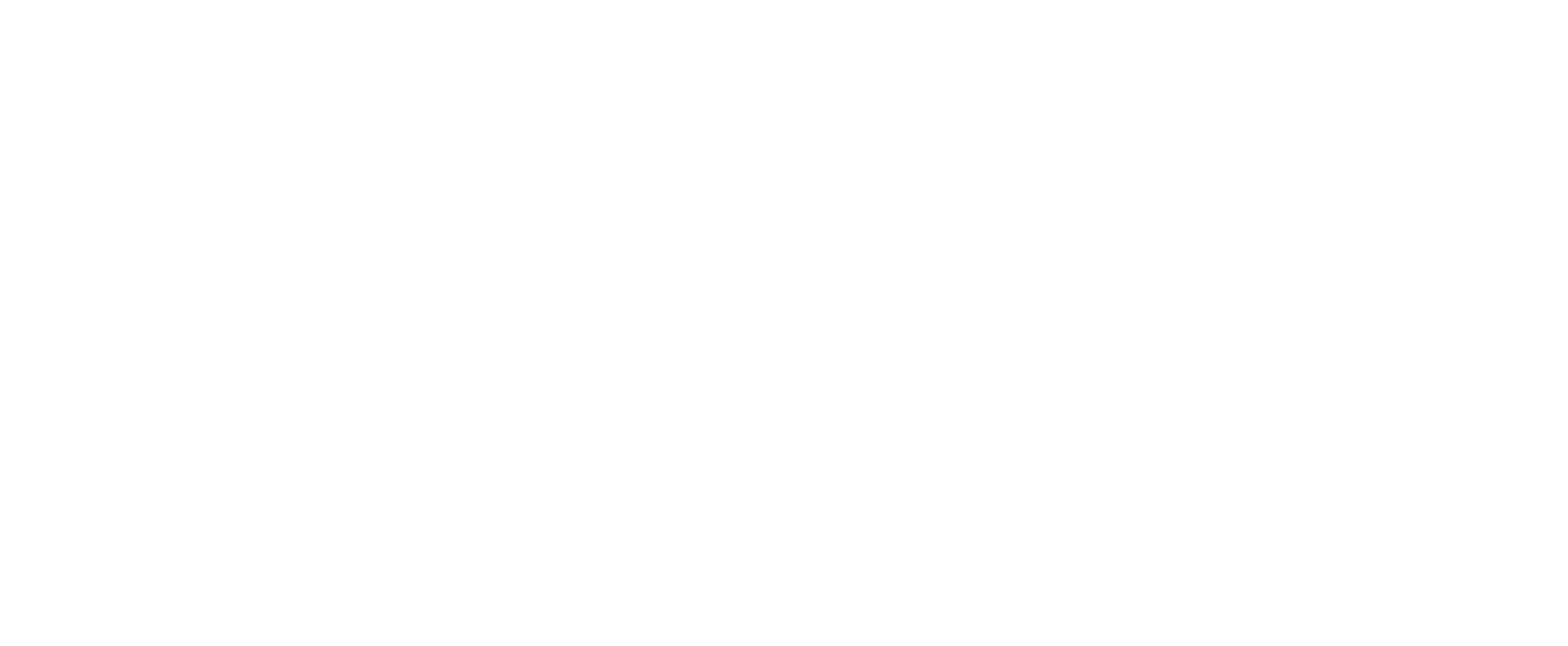•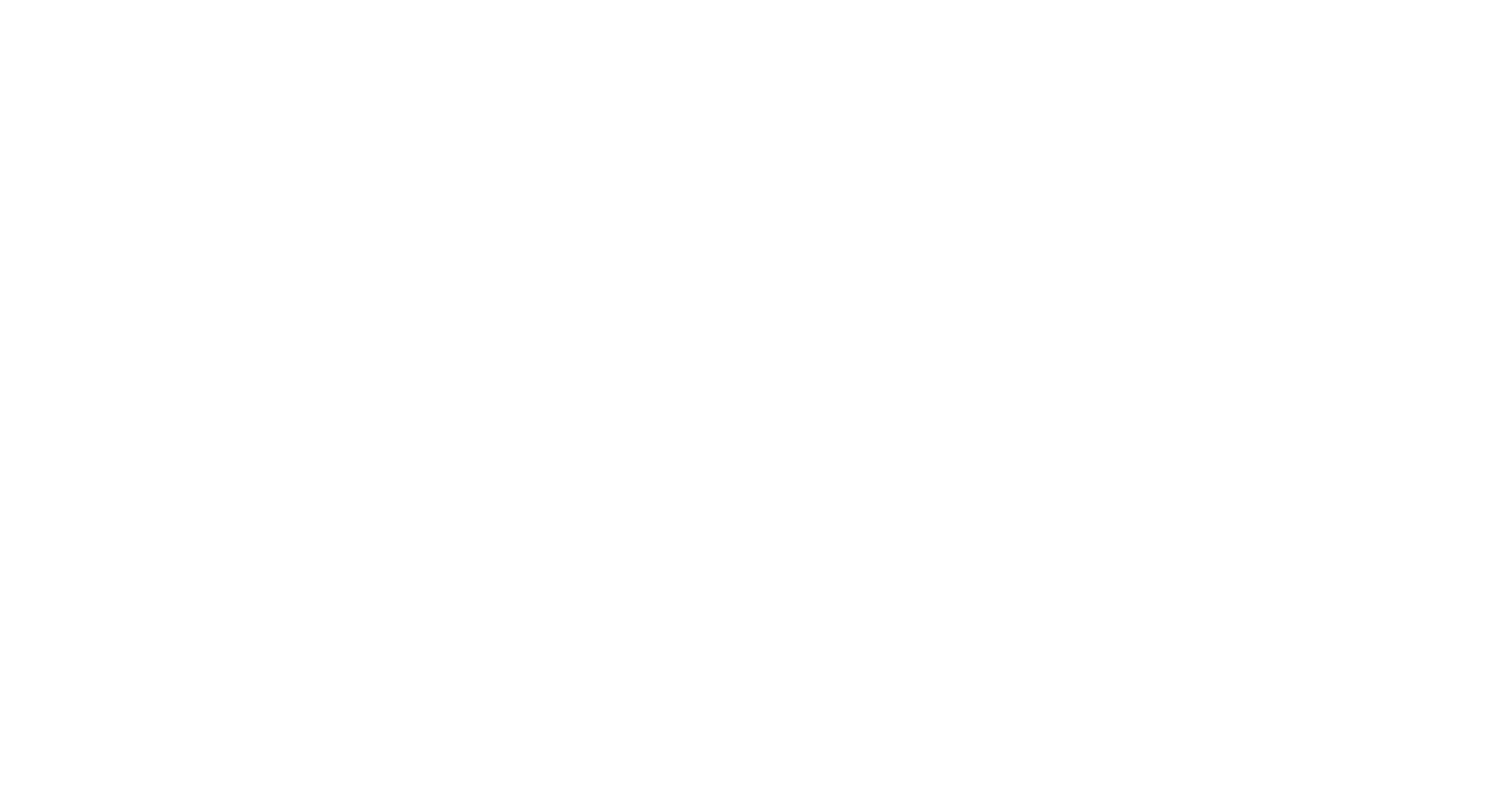•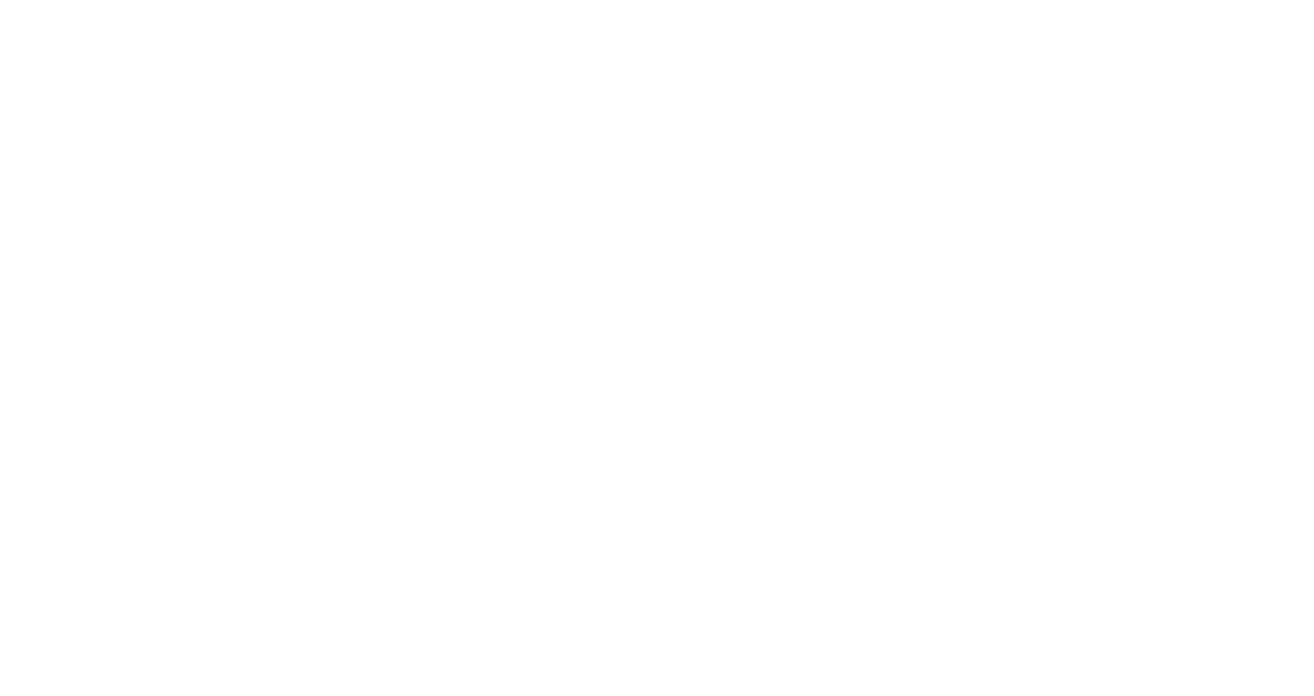Knowledge Graphs are useful to map concepts in a non-linear manner. Cheenta Genius App uses knowledge graphs to further improve student learning.

## Problems in Geometry for AMC 10

### American Mathematics contest 10 (AMC 10) - Geometry problems

AMC 10 A, 2020 , Problem 12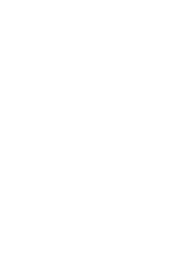Triangle $AMC$ is isosceles with $AM = AC$. Medians $\overline{MV}$ and $\overline{CU}$ are perpendicular to each other, and $MV=CU=12$. What is the area of $\triangle AMC?$

$\textbf{(A) } 48 \qquad \textbf{(B) } 72 \qquad \textbf{(C) } 96 \qquad \textbf{(D) } 144 \qquad \textbf{(E) } 192$

AMC 10A, 2020, Problem 16

A point is chosen at random within the square in the coordinate plane whose vertices are $(0, 0), (2020, 0), (2020, 2020),$ and $(0, 2020)$. The probability that the point is within $d$ units of a lattice point is $\frac{1}{2}$. (A point $(x, y)$ is a lattice point if $x$ and $y$ are both integers.) What is $d$ to the nearest tenth$?$

$\textbf{(A) } 0.3 \qquad \textbf{(B) } 0.4 \qquad \textbf{(C) } 0.5 \qquad \textbf{(D) } 0.6 \qquad \textbf{(E) } 0.7$

AMC 10A, 2020, Problem 19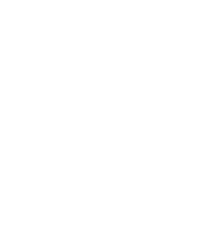As shown in the figure below, a regular dodecahedron (the polyhedron consisting of $12$ congruent regular pentagonal faces) floats in space with two horizontal faces. Note that there is a ring of five slanted faces adjacent to the top face, and a ring of five slanted faces adjacent to the bottom face. How many ways are there to move from the top face to the bottom face via a sequence of adjacent faces so that each face is visited at most once and moves are not permitted from the bottom ring to the top ring?

$\textbf{(A) } 125 \qquad \textbf{(B) } 250 \qquad \textbf{(C) } 405 \qquad \textbf{(D) } 640 \qquad \textbf{(E) } 810$

AMC 10A, 2020, Problem 20

Quadrilateral $ABCD$ satisfies $\angle ABC = \angle ACD = 90^{\circ}, AC=20,$ and $CD=30.$ Diagonals $\overline{AC}$ and $\overline{BD}$ intersect at point $E,$ and $AE=5.$ What is the area of quadrilateral $ABCD?$

$\textbf{(A) } 330 \qquad \textbf{(B) } 340 \qquad \textbf{(C) } 350 \qquad \textbf{(D) } 360 \qquad \textbf{(E) } 370$

AMC 10A, 2020, Problem 23

Let $T$ be the triangle in the coordinate plane with vertices $(0,0), (4,0),$ and $(0,3).$ Consider the following five isometries (rigid transformations) of the plane: rotations of $90^{\circ}, 180^{\circ},$ and $270^{\circ}$ counterclockwise around the origin, reflection across the $x$-axis, and reflection across the $y$-axis. How many of the $125$ sequences of three of these transformations (not necessarily distinct) will return $T$ to its original position? (For example, a $180^{\circ}$ rotation, followed by a reflection across the $x$-axis, followed by a reflection across the $y$-axis will return $T$ to its original position, but a $90^{\circ}$ rotation, followed by a reflection across the $x$-axis, followed by another reflection across the $x$-axis will not return $T$ to its original position.)

$\textbf{(A) } 12 \qquad \textbf{(B) } 15 \qquad \textbf{(C) } 17 \qquad \textbf{(D) } 20 \qquad \textbf{(E) } 25$

AMC 10A, 2019, Problem 6

For how many of the following types of quadrilaterals does there exist a point in the plane of the quadrilateral that is equidistant from all four vertices of the quadrilateral?

a square
a rectangle that is not a square
a rhombus that is not a square
a parallelogram that is not a rectangle or a rhombus
an isosceles trapezoid that is not a parallelogram
$\textbf{(A) } 1 \qquad\textbf{(B) } 2 \qquad\textbf{(C) } 3 \qquad\textbf{(D) } 4 \qquad\textbf{(E) } 5$

AMC 10A, 2019, Problem 7

Two lines with slopes $\frac{1}{2}$ and $2$ intersect at $(2,2)$. What is the area of the triangle enclosed by these two lines and the line $x+y=10 ?$

$\textbf{(A) } 4 \qquad\textbf{(B) } 4\sqrt{2} \qquad\textbf{(C) } 6 \qquad\textbf{(D) } 8 \qquad\textbf{(E) } 6\sqrt{2}$

AMC 10A, 2019, Problem 8

The figure below shows line $\ell$ with a regular, infinite, recurring pattern of squares and line segments.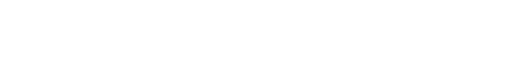How many of the following four kinds of rigid motion transformations of the plane in which this figure is drawn, other than the identity transformation, will transform this figure into itself?

some rotation around a point of line $\ell$
some translation in the direction parallel to line $\ell$
the reflection across line $\ell$
some reflection across a line perpendicular to line $\ell$
$\textbf{(A) } 0 \qquad\textbf{(B) } 1 \qquad\textbf{(C) } 2 \qquad\textbf{(D) } 3 \qquad\textbf{(E) } 4$

AMC 10A, 2019, Problem 10

A rectangular floor that is $10$ feet wide and $17$ feet long is tiled with $170$ one-foot square tiles. A bug walks from one corner to the opposite corner in a straight line. Including the first and the last tile, how many tiles does the bug visit?

$\textbf{(A) } 17 \qquad\textbf{(B) } 25 \qquad\textbf{(C) } 26 \qquad\textbf{(D) } 27 \qquad\textbf{(E) } 28$

AMC 10A, 2019, Problem 13

Let $\triangle ABC$ be an isosceles triangle with $BC = AC$ and $\angle ACB = 40^{\circ}$. Construct the circle with diameter $\overline{BC}$, and let $D$ and $E$ be the other intersection points of the circle with the sides $\overline{AC}$ and $\overline{AB}$, respectively. Let $F$ be the intersection of the diagonals of the quadrilateral $BCDE$. What is the degree measure of $\angle BFC ?$

$\textbf{(A) } 90 \qquad\textbf{(B) } 100 \qquad\textbf{(C) } 105 \qquad\textbf{(D) } 110 \qquad\textbf{(E) } 120$

AMC 10A, 2019, Problem 16

The figure below shows $13$ circles of radius $1$ within a larger circle. All the intersections occur at points of tangency. What is the area of the region, shaded in the figure, inside the larger circle but outside all the circles of radius $1 ?$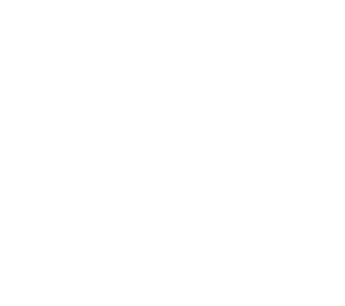$\textbf{(A) } 4 \pi \sqrt{3} \qquad\textbf{(B) } 7 \pi \qquad\textbf{(C) } \pi\left(3\sqrt{3} +2\right) \qquad\textbf{(D) } 10 \pi \left(\sqrt{3} - 1\right) \qquad\textbf{(E) } \pi\left(\sqrt{3} + 6\right)$

AMC 10A, 2019, Problem 21

A sphere with center $O$ has radius 6. A triangle with sides of length $15$, $15$, and $24$ is situated in space so that each of its sides are tangent to the sphere. What is the distance between $O$ and the plane determined by the triangle?

$\textbf{(A) } 2\sqrt{3} \qquad \textbf{(B) }4 \qquad \textbf{(C) } 3\sqrt{2} \qquad \textbf{(D) } 2\sqrt{5} \qquad \textbf{(E) } 5$

AMC 10B, 2019, Problem 5

Triangle $ABC$ lies in the first quadrant. Points $A$, $B$, and $C$ are reflected across the line $y=x$ to points $A'$, $B'$, and $C'$, respectively. Assume that none of the vertices of the triangle lie on the line $y=x$. Which of the following statements is not always true?

$\textbf{(A) }$ Triangle $A'B'C'$ lies in the first quadrant.

$\textbf{(B) }$ Triangles $ABC$ and $A'B'C'$ have the same area.

$\textbf{(C) }$ The slope of line $AA'$ is $-1$.

$\textbf{(D) }$ The slopes of lines $AA'$ and $CC'$ are the same.

$\textbf{(E) }$ Lines $AB$ and $A'B'$ are perpendicular to each other

AMC 10B, 2019, Problem 8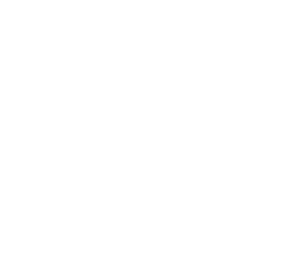In triangle $ABC$, point $D$ divides side $\overline{AC}$ so that $AD:DC=1:2$. Let $E$ be the midpoint of $\overline{BD}$ and let $F$ be the point of intersection of line $BC$ and line $AE$. Given that the area of $\triangle ABC$ is $360$, what is the area of $\triangle EBF$?

AMC 10B, 2019, Problem 15

Right triangles $T_1$ and $T_2$ have areas 1 and 2, respectively. A side of $T_1$ is congruent to a side of $T_2$, and a different side of $T_1$ is congruent to a different side of $T_2$. What is the square of the product of the other (third) sides of $T_1$ and $T_2$?

$\textbf{(A) } \frac{28}{3} \qquad\textbf{(B) }10\qquad\textbf{(C) } \frac{32}{3} \qquad\textbf{(D) } \frac{34}{3} \qquad\textbf{(E) }12$

AMC 10B, 2019, Problem 16

In $\triangle ABC$ with a right angle at $C,$ point $D$ lies in the interior of $\overline{AB}$ and point $E$ lies in the interior of $\overline{BC}$ so that $AC=CD,$ $DE=EB,$ and the ratio $AC:DE=4:3.$ What is the ratio $AD:DB?$

$\textbf{(A) } 2:3 \qquad\textbf{(B) } 2:\sqrt{5} \qquad\textbf{(C) } 1:1 \qquad\textbf{(D) } 3:\sqrt{5} \qquad\textbf{(E) } 3:2$

AMC 10B, 2019, Problem 20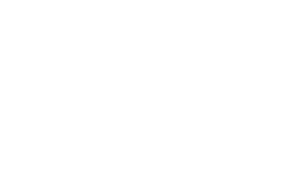As shown in the figure, line segment $\overline{AD}$ is trisected by points $B$ and $C$ so that $AB=BC=CD=2$. Three semicircles of radius $1$,$\overparen{AEB},\overparen{BFC},$ and $\overparen{CGD}$ , have their diameters on $\overline{AD}$, and are tangent to line $EG$ at $E,F,$ and $G,$ respectively. A circle of radius $2$ has its center on $F.$ The area of the region inside the circle but outside the three semicircles, shaded in the figure, can be expressed in the form             $\frac{a}{b}\cdot\pi-\sqrt{c}+d,$where $a,b,c,$ and $d$ are positive integers and $a$ and $b$ are relatively prime. What is $a+b+c+d$?

AMC 10B, 2019, Problem 23

Points $A(6,13)$ and $B(12,11)$ lie on circle $\omega$ in the plane. Suppose that the tangent lines to $\omega$ at $A$ and $B$ intersect at a point on the $x$-axis. What is the area of $\omega$?

$\textbf{(A) }\frac{83\pi}{8}\qquad\textbf{(B) }\frac{21\pi}{2}\qquad\textbf{(C) } \frac{85\pi}{8}\qquad\textbf{(D) }\frac{43\pi}{4}\qquad\textbf{(E) }\frac{87\pi}{8}$

AMC 10A, 2018, Problem 9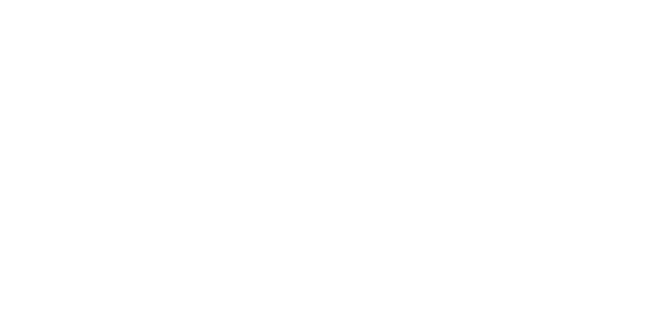All of the triangles in the diagram below are similar to isosceles triangle $ABC$, in which $AB=AC$. Each of the 7 smallest triangles has area 1, and $\triangle ABC$ has area 40. What is the area of trapezoid $DBCE$?

$\textbf{(A) } 16 \qquad \textbf{(B) } 18 \qquad \textbf{(C) } 20 \qquad \textbf{(D) } 22 \qquad \textbf{(E) } 24$

AMC 10A, 2018, Problem 13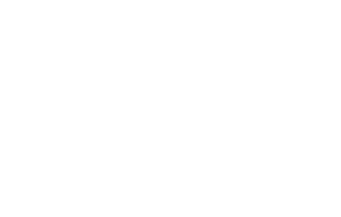A paper triangle with sides of lengths 3, 4, and 5 inches, as shown, is folded so that point $A$ falls on point $B$. What is the length in inches of the crease?

$\textbf{(A) } 1+\frac12 \sqrt2 \qquad \textbf{(B) } \sqrt3 \qquad \textbf{(C) } \frac74 \qquad \textbf{(D) } \frac{15}{8} \qquad \textbf{(E) } 2$

AMC 10A, 2018, Problem 15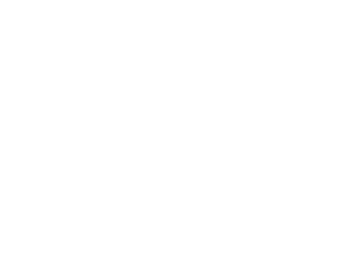Two circles of radius 5 are externally tangent to each other and are internally tangent to a circle of radius 13 at points $A$ and $B$, as shown in the diagram. The distance $AB$ can be written in the form $\frac{m}{n}$, where $m$ and $n$ are relatively prime positive integers. What is $m+n$?

$\textbf{(A) } 21 \qquad \textbf{(B) } 29 \qquad \textbf{(C) } 58 \qquad \textbf{(D) } 69 \qquad \textbf{(E) } 93$

AMC 10A, 2018, Problem 16

Right triangle $ABC$ has leg lengths $AB=20$ and $BC=21$. Including $\overline{AB}$ and $\overline{BC}$, how many line segments with integer length can be drawn from vertex $B$ to a point on hypotenuse $\overline{AC}$?

$\textbf{(A) }5 \qquad \textbf{(B) }8 \qquad \textbf{(C) }12 \qquad \textbf{(D) }13 \qquad \textbf{(E) }15 \qquad$

AMC 10A, 2018, Problem 20

A scanning code consists of a $7 \times 7$ grid of squares, with some of its squares colored black and the rest colored white. There must be at least one square of each color in this grid of $49$ squares. A scanning code is called $\text{symmetric}$ if its look does not change when the entire square is rotated by a multiple of $90 ^{\circ}$ counterclockwise around its center, nor when it is reflected across a line joining opposite corners or a line joining midpoints of opposite sides. What is the total number of possible symmetric scanning codes?

$\textbf{(A)} \text{ 510} \qquad \textbf{(B)} \text{ 1022} \qquad \textbf{(C)} \text{ 8190} \qquad \textbf{(D)} \text{ 8192} \qquad \textbf{(E)} \text{ 65,534}$

AMC 10A, 2018, Problem 23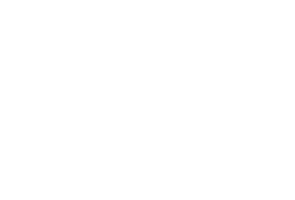Farmer Pythagoras has a field in the shape of a right triangle. The right triangle's legs have lengths 3 and 4 units. In the corner where those sides meet at a right angle, he leaves a small unplanted square $S$ so that from the air it looks like the right angle symbol. The rest of the field is planted. The shortest distance from $S$ to the hypotenuse is 2 units. What fraction of the field is planted?

$\textbf{(A) } \frac{25}{27} \qquad \textbf{(B) } \frac{26}{27} \qquad \textbf{(C) } \frac{73}{75} \qquad \textbf{(D) } \frac{145}{147} \qquad \textbf{(E) } \frac{74}{75}$

AMC 10A, 2018, Problem 24

Triangle $ABC$ with $AB=50$ and $AC=10$ has area $120$. Let $D$ be the midpoint of $\overline{AB}$, and let $E$ be the midpoint of $\overline{AC}$. The angle bisector of $\angle BAC$ intersects $\overline{DE}$ and $\overline{BC}$ at $F$ and $G$, respectively. What is the area of quadrilateral $FDBG$?

$\textbf{(A) }60 \qquad \textbf{(B) }65 \qquad \textbf{(C) }70 \qquad \textbf{(D) }75 \qquad \textbf{(E) }80 \qquad$

AMC 10B, 2018, Problem 4

A three-dimensional rectangular box with dimensions $X$, $Y$, and $Z$ has faces whose surface areas are $24, 24, 48, 48, 72,$ and $72$ square units. What is $X+Y+Z$?

$\textbf{(A) }18 \qquad \textbf{(B) }22 \qquad \textbf{(C) }24 \qquad \textbf{(D) }30 \qquad \textbf{(E) }36 \qquad$

AMC 10B, 2018, Problem 7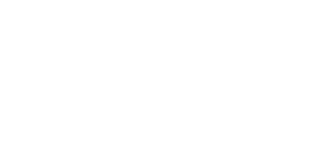In the figure below, $N$ congruent semicircles are drawn along a diameter of a large semicircle, with their diameters covering the diameter of the large semicircle with no overlap. Let $A$ be the combined area of the small semicircles and $B$ be the area of the region inside the large semicircle but outside the small semicircles. The ratio $A:B$ is $1:18$. What is $N$?

$\textbf{(A) }16 \qquad \textbf{(B) }17 \qquad \textbf{(C) }18 \qquad \textbf{(D) }19 \qquad \textbf{(E) }36 \qquad$

AMC 10B, 2018, Problem 10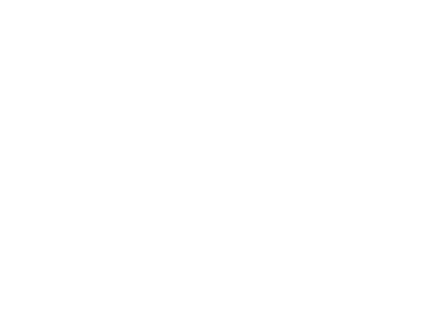In the rectangular parallelepiped shown, $AB=3$, $BC=1$, and $CG=2$. Point $M$ is the midpoint of $\overline{FG}$. What is the volume of the rectangular pyramid with base $BCHE$ and apex $M$?

$\textbf{(A) }1 \qquad \textbf{(B) }\frac{4}{3} \qquad \textbf{(C) }\frac{3}{2} \qquad \textbf{(D) }\frac{5}{3} \qquad \textbf{(E) }2 \qquad$

AMC 10B, 2018, Problem 12

Line segment $\overline{AB}$ is a diameter of a circle with $AB=24$. Point $C$, not equal to $A$ or $B$, lies on the circle. As point $C$ moves around the circle, the centroid (center of mass) of $\triangle{ABC}$ traces out a closed curve missing two points. To the nearest positive integer, what is the area of the region bounded by this curve?

$\textbf{(A) }25 \qquad \textbf{(B) }38 \qquad \textbf{(C) }50 \qquad \textbf{(D) }63 \qquad \textbf{(E) }75 \qquad$

AMC 10B, 2018, Problem 15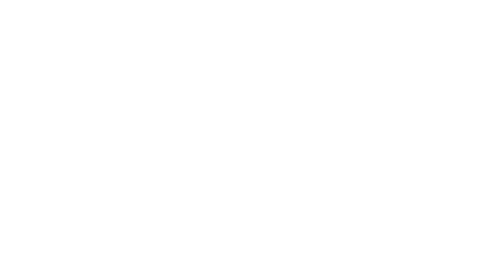A closed box with a square base is to be wrapped with a square sheet of wrapping paper. The box is centered on the wrapping paper with the vertices of the base lying on the midlines of the square sheet of paper, as shown in the figure on the left. The four corners of the wrapping paper are to be folded up over the sides and brought together to meet at the center of the top of the box, point $A$ in the figure on the right. The box has base length $w$ and height $h$. What is the area of the sheet of wrapping paper?

$\textbf{(A) } 2(w+h)^2 \qquad \textbf{(B) } \frac{(w+h)^2}2 \qquad \textbf{(C) } 2w^2+4wh \qquad \textbf{(D) } 2w^2 \qquad \textbf{(E) } w^2h$

AMC 10B, 2018, Problem 17

In rectangle $PQRS$, $PQ=8$ and $QR=6$. Points $A$ and $B$ lie on $\overline{PQ}$, points $C$ and $D$ lie on $\overline{QR}$, points $E$ and $F$ lie on $\overline{RS}$, and points $G$ and $H$ lie on $\overline{SP}$ so that $AP=BQ<4$ and the convex octagon $ABCDEFGH$ is equilateral. The length of a side of this octagon can be expressed in the form $k+m\sqrt{n}$, where $k$, $m$, and $n$ are integers and $n$ is not divisible by the square of any prime. What is $k+m+n$?

$\textbf{(A) }1 \qquad \textbf{(B) }7 \qquad \textbf{(C) }21 \qquad \textbf{(D) }92 \qquad \textbf{(E) }106 \qquad$

AMC 10B, 2018, Problem 24

Let $ABCDEF$ be a regular hexagon with side length $1$. Denote by $X$, $Y$, and $Z$ the midpoints of sides $\overline {AB}$, $\overline{CD}$, and $\overline{EF}$, respectively. What is the area of the convex hexagon whose interior is the intersection of the interiors of $\triangle ACE$ and $\triangle XYZ$?

$\textbf{(A)} \frac {3}{8}\sqrt{3} \qquad \textbf{(B)} \frac {7}{16}\sqrt{3} \qquad \textbf{(C)} \frac {15}{32}\sqrt{3} \qquad \textbf{(D)} \frac {1}{2}\sqrt{3} \qquad \textbf{(E)} \frac {9}{16}\sqrt{3} \qquad$

AMC 10A, 2017, Problem 3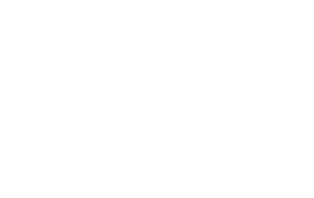Amara has three rows of two $6$-feet by $2$-feet flower beds in her garden. The beds are separated and also surrounded by $1$-foot-wide walkways, as shown on the diagram. What is the total area of the walkways, in square feet?

$\textbf{(A)}\ 72\qquad\textbf{(B)}\ 78\qquad\textbf{(C)}\ 90\qquad\textbf{(D)}\ 120\qquad\textbf{(E)}\ 150$

AMC 10A, 2017, Problem 21

A square with side length $x$ is inscribed in a right triangle with sides of length $3$, $4$, and $5$ so that one vertex of the square coincides with the right-angle vertex of the triangle. A square with side length $y$ is inscribed in another right triangle with sides of length $3$, $4$, and $5$ so that one side of the square lies on the hypotenuse of the triangle. What is $\frac{x}{y}$?

$\textbf{(A) } \frac{12}{13} \qquad \textbf{(B) } \frac{35}{37} \qquad \textbf{(C) } 1 \qquad \textbf{(D) } \frac{37}{35} \qquad \textbf{(E) } \frac{13}{12}$

AMC 10A, 2017, Problem 22

Sides $\overline{AB}$ and $\overline{AC}$ of equilateral triangle $ABC$ are tangent to a circle at points $B$ and $C$ respectively. What fraction of the area of $\triangle ABC$ lies outside the circle?

$\textbf{(A)}\ \frac{4\sqrt{3}\pi}{27}-\frac{1}{3}\qquad\textbf{(B)}\ \frac{\sqrt{3}}{2}-\frac{\pi}{8}\qquad\textbf{(C)}\ \frac{1}{2}\qquad\textbf{(D)}\ \sqrt{3}-\frac{2\sqrt{3}\pi}{9}\qquad\textbf{(E)}\ \frac{4}{3}-\frac{4\sqrt{3}\pi}{27}$

AMC 10A, 2017, Problem 23

How many triangles with positive area have all their vertices at points $(i,j)$ in the coordinate plane, where $i$ and $j$ are integers between $1$ and $5$, inclusive?

$\textbf{(A)}\ 2128 \qquad\textbf{(B)}\ 2148 \qquad\textbf{(C)}\ 2160 \qquad\textbf{(D)}\ 2200 \qquad\textbf{(E)}\ 2300$

AMC 10B, 2017, Problem 6

What is the largest number of solid $2\text{ in.}$ by $2\text{ in.}$ by $1\text{ in.}$ blocks that can fit in a $3\text{ in.}$ by $2\text{ in.}$ by $3\text{ in.}$ box?

$\textbf{(A)}\ 3\qquad\textbf{(B)}\ 4\qquad\textbf{(C)}\ 5\qquad\textbf{(D)}\ 6\qquad\textbf{(E)}\ 7$

AMC 10B, 2017, Problem 8

Points $A(11, 9)$ and $B(2, -3)$ are vertices of $\triangle ABC$ with $AB=AC$. The altitude from $A$ meets the opposite side at $D(-1, 3)$. What are the coordinates of point $C$?

$\textbf{(A)}\ (-8, 9)\qquad\textbf{(B)}\ (-4, 8)\qquad\textbf{(C)}\ (-4, 9)\qquad\textbf{(D)}\ (-2, 3)\qquad\textbf{(E)}\ (-1, 0)$

AMC 10B, 2017, Problem 15

Rectangle $ABCD$ has $AB=3$ and $BC=4$. Point $E$ is the foot of the perpendicular from $B$ to diagonal $\overline{AC}$. What is the area of $\triangle AED$?

$\textbf{(A)}\ 1\qquad\textbf{(B)}\ \frac{42}{25}\qquad\textbf{(C)}\ \frac{28}{15}\qquad\textbf{(D)}\ 2\qquad\textbf{(E)}\ \frac{54}{25}$

AMC 10B, 2017, Problem 19

Let $ABC$ be an equilateral triangle. Extend side $\overline{AB}$ beyond $B$ to a point $B'$ so that $BB'=3AB$. Similarly, extend side $\overline{BC}$ beyond $C$ to a point $C'$ so that $CC'=3BC$, and extend side $\overline{CA}$ beyond $A$ to a point $A'$ so that $AA'=3CA$. What is the ratio of the area of $\triangle A'B'C'$ to the area of $\triangle ABC$?

$\textbf{(A)}\ 9:1\qquad\textbf{(B)}\ 16:1\qquad\textbf{(C)}\ 25:1\qquad\textbf{(D)}\ 36:1\qquad\textbf{(E)}\ 37:1$ AMC 10B, 2017, Problem 21 In $\triangle ABC$, $AB=6$, $AC=8$, $BC=10$, and $D$ is the midpoint of $\overline{BC}$. What is the sum of the radii of the circles inscribed in $\triangle ADB$ and $\triangle ADC$?

$\textbf{(A)}\ \sqrt{5}\qquad\textbf{(B)}\ \frac{11}{4}\qquad\textbf{(C)}\ 2\sqrt{2}\qquad\textbf{(D)}\ \frac{17}{6}\qquad\textbf{(E)}\ 3$

AMC 10B, 2017, Problem 22

The diameter $AB$ of a circle of radius $2$ is extended to a point $D$ outside the circle so that $BD=3$. Point $E$ is chosen so that $ED=5$ and line $ED$ is perpendicular to line $AD$. Segment $AE$ intersects the circle at a point $C$ between $A$ and $E$. What is the area of $\triangle ABC$?

$\textbf{(A)}\ \frac{120}{37}\qquad\textbf{(B)}\ \frac{140}{39}\qquad\textbf{(C)}\ \frac{145}{39}\qquad\textbf{(D)}\ \frac{140}{37}\qquad\textbf{(E)}\ \frac{120}{31}$

AMC 10B, 2017, Problem 24

The vertices of an equilateral triangle lie on the hyperbola $xy=1$, and a vertex of this hyperbola is the centroid of the triangle. What is the square of the area of the triangle?

$\textbf{(A)}\ 48\qquad\textbf{(B)}\ 60\qquad\textbf{(C)}\ 108\qquad\textbf{(D)}\ 120\qquad\textbf{(E)}\ 169$

AMC 10A, 2016, Problem 5

A rectangular box has integer side lengths in the ratio $1: 3: 4$. Which of the following could be the volume of the box?

$\textbf{(A)}\ 48\qquad\textbf{(B)}\ 56\qquad\textbf{(C)}\ 64\qquad\textbf{(D)}\ 96\qquad\textbf{(E)}\ 144$

AMC 10A, 2016, Problem 10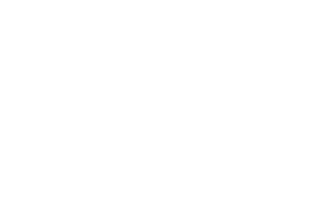A rug is made with three different colors as shown. The areas of the three differently colored regions form an arithmetic progression. The inner rectangle is one foot wide, and each of the two shaded regions is $1$ foot wide on all four sides. What is the length in feet of the inner rectangle?

$\textbf{(A) } 1 \qquad \textbf{(B) } 2 \qquad \textbf{(C) } 4 \qquad \textbf{(D) } 6 \qquad \textbf{(E) }8$

AMC 10A, 2016, Problem 11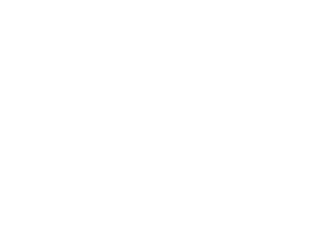Find the area of the shaded region.

$\textbf{(A)}\ 4\frac{3}{5} \qquad \textbf{(B)}\ 5\qquad \textbf{(C)}\ 5\frac{1}{4} \qquad \textbf{(D)}\ 6\frac{1}{2} \qquad \textbf{(E)}\ 8$

AMC 10A, 2016, Problem 15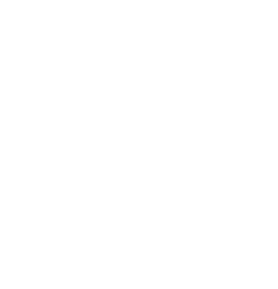Seven cookies of radius $1$ inch are cut from a circle of cookie dough, as shown. Neighboring cookies are tangent, and all except the center cookie are tangent to the edge of the dough. The leftover scrap is reshaped to form another cookie of the same thickness. What is the radius in inches of the scrap cookie?

$\textbf{(A) } \sqrt{2} \qquad \textbf{(B) } 1.5 \qquad \textbf{(C) } \sqrt{\pi} \qquad \textbf{(D) } \sqrt{2\pi} \qquad \textbf{(E) } \pi$

AMC 10A, 2016, Problem 16

A triangle with vertices $A(0, 2)$, $B(-3, 2)$, and $C(-3, 0)$ is reflected about the $x$-axis, then the image $\triangle A'B'C'$ is rotated counterclockwise about the origin by $90^{\circ}$ to produce $\triangle A''B''C''$. Which of the following transformations will return $\triangle A''B''C''$ to $\triangle ABC$?

$\textbf{(A)}$ counterclockwise rotation about the origin by $90^{\circ}$.

$\textbf{(B)}$ clockwise rotation about the origin by $90^{\circ}$.

$\textbf{(C)}$ reflection about the $x$-axis

$\textbf{(D)}$ reflection about the line $y = x$

$\textbf{(E)}$ reflection about the $y$-axis.

AMC 10A, 2016, Problem 18

Each vertex of a cube is to be labeled with an integer $1$ through $8$, with each integer being used once, in such a way that the sum of the four numbers on the vertices of a face is the same for each face. Arrangements that can be obtained from each other through rotations of the cube are considered to be the same. How many different arrangements are possible?

$\textbf{(A) } 1\qquad\textbf{(B) } 3\qquad\textbf{(C) }6 \qquad\textbf{(D) }12 \qquad\textbf{(E) }24$

AMC 10A, 2016, Problem 19

In rectangle $ABCD,$ $AB=6$ and $BC=3$. Point $E$ between $B$ and $C$, and point $F$ between $E$ and $C$ are such that $BE=EF=FC$. Segments $\overline{AE}$ and $\overline{AF}$ intersect $\overline{BD}$ at $P$ and $Q$, respectively. The ratio $BP:PQ:QD$ can be written as $r:s:t$ where the greatest common factor of $r,s$ and $t$ is 1. What is $r+s+t$?

$\textbf{(A) } 7 \qquad \textbf{(B) } 9 \qquad \textbf{(C) } 12 \qquad \textbf{(D) } 15 \qquad \textbf{(E) } 20$

AMC 10A, 2016, Problem 21

Circles with centers $P, Q$ and $R$, having radii $1, 2$ and $3$, respectively, lie on the same side of line $l$ and are tangent to $l$ at $P', Q'$ and $R'$, respectively, with $Q'$ between $P'$ and $R'$. The circle with center $Q$ is externally tangent to each of the other two circles. What is the area of triangle $PQR$?

$\textbf{(A) } 0\qquad \textbf{(B) } \sqrt{\frac{2}{3}}\qquad\textbf{(C) } 1\qquad\textbf{(D) } \sqrt{6}-\sqrt{2}\qquad\textbf{(E) }\sqrt{\frac{3}{2}}$

AMC 10A, 2016, Problem 24

A quadrilateral is inscribed in a circle of radius $200\sqrt{2}$. Three of the sides of this quadrilateral have length $200$. What is the length of the fourth side?

$\textbf{(A) }200\qquad \textbf{(B) }200\sqrt{2}\qquad\textbf{(C) }200\sqrt{3}\qquad\textbf{(D) }300\sqrt{2}\qquad\textbf{(E) } 500$

AMC 10B, 2016, Problem 7

The ratio of the measures of two acute angles is $5:4$, and the complement of one of these two angles is twice as large as the complement of the other. What is the sum of the degree measures of the two angles?

$\textbf{(A)}\ 75\qquad\textbf{(B)}\ 90\qquad\textbf{(C)}\ 135\qquad\textbf{(D)}\ 150\qquad\textbf{(E)}\ 270$

AMC 10B, 2016, Problem 9

All three vertices of $\bigtriangleup ABC$ are lying on the parabola defined by $y=x^2$, with $A$ at the origin and $\overline{BC}$ parallel to the $x$-axis. The area of the triangle is $64$. What is the length of $BC$?

$\textbf{(A)}\ 4\qquad\textbf{(B)}\ 6\qquad\textbf{(C)}\ 8\qquad\textbf{(D)}\ 10\qquad\textbf{(E)}\ 16$

AMC 10B, 2016, Problem 10

A thin piece of wood of uniform density in the shape of an equilateral triangle with side length $3$ inches weighs $12$ ounces. A second piece of the same type of wood, with the same thickness, also in the shape of an equilateral triangle, has side length of $5$ inches. Which of the following is closest to the weight, in ounces, of the second piece?

$\textbf{(A)}\ 14.0\qquad\textbf{(B)}\ 16.0\qquad\textbf{(C)}\ 20.0\qquad\textbf{(D)}\ 33.3\qquad\textbf{(E)}\ 55.6$

AMC 10B, 2016, Problem 11

Carl decided to fence in his rectangular garden. He bought $20$ fence posts, placed one on each of the four corners, and spaced out the rest evenly along the edges of the garden, leaving exactly $4$ yards between neighboring posts. The longer side of his garden, including the corners, has twice as many posts as the shorter side, including the corners. What is the area, in square yards, of Carl’s garden?

$\textbf{(A)}\ 256\qquad\textbf{(B)}\ 336\qquad\textbf{(C)}\ 384\qquad\textbf{(D)}\ 448\qquad\textbf{(E)}\ 512$

AMC 10B, 2016, Problem 14

How many squares whose sides are parallel to the axis and whose vertices have coordinates that are integers lie entirely within the region bounded by the line $y=\pi x$, the line $y=-0.1$ and the line $x=5.1?$

$\textbf{(A)}\ 30 \qquad \textbf{(B)}\ 41 \qquad \textbf{(C)}\ 45 \qquad \textbf{(D)}\ 50 \qquad \textbf{(E)}\ 57$

AMC 10B, 2016, Problem 16

The sum of an infinite geometric series is a positive number $S$, and the second term in the series is $1$. What is the smallest possible value of $S?$

$\textbf{(A)}\ \frac{1+\sqrt{5}}{2} \qquad \textbf{(B)}\ 2 \qquad \textbf{(C)}\ \sqrt{5} \qquad \textbf{(D)}\ 3 \qquad \textbf{(E)}\ 4$

AMC 10B, 2016, Problem 19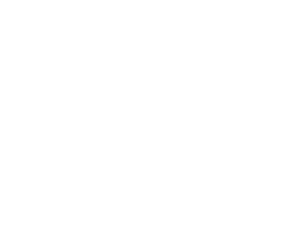Rectangle $ABCD$ has $AB=5$ and $BC=4$. Point $E$ lies on $\overline{AB}$ so that $EB=1$, point $G$ lies on $\overline{BC}$ so that $CG=1$. and point $F$ lies on $\overline{CD}$ so that $DF=2$. Segments $\overline{AG}$ and $\overline{AC}$ intersect $\overline{EF}$ at $Q$ and $P$, respectively. What is the value of $\frac{PQ}{EF}$?

$\textbf{(A)}~\frac{\sqrt{13}}{16} \qquad \textbf{(B)}~\frac{\sqrt{2}}{13} \qquad \textbf{(C)}~\frac{9}{82} \qquad \textbf{(D)}~\frac{10}{91}\qquad \textbf{(E)}~\frac19$

AMC 10B, 2016, Problem 20

A dilation of the plane—that is, a size transformation with a positive scale factor—sends the circle of radius $2$ centered at $A(2,2)$ to the circle of radius $3$ centered at $A’(5,6)$. What distance does the origin $O(0,0)$, move under this transformation?

$\textbf{(A)}\ 0\qquad\textbf{(B)}\ 3\qquad\textbf{(C)}\ \sqrt{13}\qquad\textbf{(D)}\ 4\qquad\textbf{(E)}\ 5$

AMC 10B, 2016, Problem 21

What is the area of the region enclosed by the graph of the equation $x^2+y^2=|x|+|y|?$

$\textbf{(A)}\ \pi+\sqrt{2}\qquad\textbf{(B)}\ \pi+2\qquad\textbf{(C)}\ \pi+2\sqrt{2}\qquad\textbf{(D)}\ 2\pi+\sqrt{2}\qquad\textbf{(E)}\ 2\pi+2\sqrt{2}$

AMC 10B, 2016, Problem 23

In regular hexagon $ABCDEF$, points $W$, $X$, $Y$, and $Z$ are chosen on sides $\overline{BC}$, $\overline{CD}$, $\overline{EF}$, and $\overline{FA}$ respectively, so lines $AB$, $ZW$, $YX$, and $ED$ are parallel and equally spaced. What is the ratio of the area of hexagon $WCXYFZ$ to the area of hexagon $ABCDEF$?

$\textbf{(A)}\ \frac{1}{3}\qquad\textbf{(B)}\ \frac{10}{27}\qquad\textbf{(C)}\ \frac{11}{27}\qquad\textbf{(D)}\ \frac{4}{9}\qquad\textbf{(E)}\ \frac{13}{27}$

AMC 10A, 2015, Problem 11

The ratio of the length to the width of a rectangle is $4$ : $3$. If the rectangle has diagonal of length $d$, then the area may be expressed as $kd^2$ for some constant $k$. What is $k$?

$\textbf{(A)}\ \frac{2}{7}\qquad\textbf{(B)}\ \frac{3}{7}\qquad\textbf{(C)}\ \frac{12}{25}\qquad\textbf{(D)}\ \frac{16}{25}\qquad\textbf{(E)}\ \frac{3}{4}$

AMC 10A, 2015, Problem 12

Points $(\sqrt{\pi}, a)$ and $(\sqrt{\pi}, b)$ are distinct points on the graph of $y^2+x^4=2x^2y+1$. What is $|a-b|$?

$\textbf{(A) }1\qquad\textbf{(B) }\frac{\pi}{2}\qquad\textbf{(C) }2\qquad\textbf{(D) }\sqrt{1+\pi}\qquad\textbf{(E) }1+\sqrt{\pi}$

AMC 10A, 2015, Problem 17

A line that passes through the origin intersects both the line $x=1$ and the line $y=1+\frac{\sqrt{3}}{3}x$. The three lines create an equilateral triangle. What is the perimeter of the triangle?

$\textbf{(A) }2\sqrt{6}\qquad\textbf{(B) }2+2\sqrt{3}\qquad\textbf{(C) }6\qquad\textbf{(D) }3+2\sqrt{3}\qquad\textbf{(E) }6+\frac{\sqrt{3}}{3}$

AMC 10A, 2015, Problem 19

The isosceles right triangle $ABC$ has right angle at $C$ and area $12.5$. The rays trisecting $\angle ACB$ intersect $AB$ at $D$ and $E$. What is the area of $\bigtriangleup CDE$?

$\textbf{(A) }\frac{5\sqrt{2}}{3}\qquad\textbf{(B) }\frac{50\sqrt{3}-75}{4}\qquad\textbf{(C) }\frac{15\sqrt{3}}{8}\qquad\textbf{(D) }\frac{50-25\sqrt{3}}{2}\qquad\textbf{(E) }\frac{25}{6}$

AMC 10A, 2015, Problem 21

Tetrahedron $ABCD$ has $AB=5$, $AC=3$, $BC=4$, $BD=4$, $AD=3$, and $CD=\frac{12}5\sqrt2$. What is the volume of the tetrahedron?

$\textbf{(A) }3\sqrt2\qquad\textbf{(B) }2\sqrt5\qquad\textbf{(C) }\frac{24}5\qquad\textbf{(D) }3\sqrt3\qquad\textbf{(E) }\frac{24}5\sqrt2$

AMC 10B, 2015, Problem 8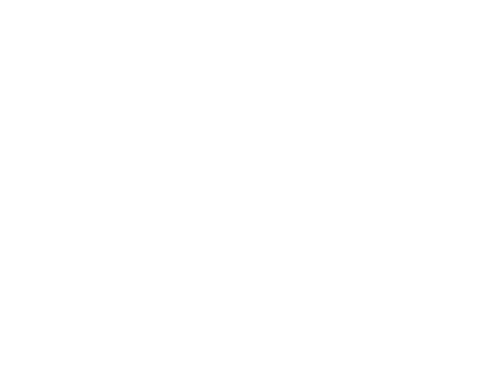The letter F shown below is rotated $90^\circ$ clockwise around the origin, then reflected in the $y$-axis, and then rotated a half turn around the origin. What is the final image?

AMC 10B, 2015, Problem 12

For how many integers $x$ is the point $(x,-x)$ inside or on the circle of radius $10$ centered at $(5,5)$?

$\textbf{(A) } 11 \qquad\textbf{(B) } 12 \qquad\textbf{(C) } 13 \qquad\textbf{(D) } 14 \qquad\textbf{(E) } 15$

AMC 10B, 2015, Problem 13

The line $12x+5y=60$ forms a triangle with the coordinate axes. What is the sum of the lengths of the altitudes of this triangle?

$\textbf{(A) } 20 \qquad\textbf{(B) } \frac{360}{17} \qquad\textbf{(C) } \frac{107}{5} \qquad\textbf{(D) } \frac{43}{2} \qquad\textbf{(E) } \frac{281}{13}$

AMC 10B, 2015, Problem 17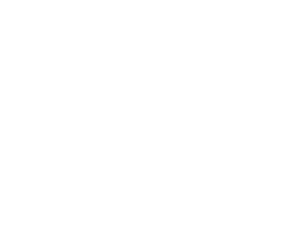When the centers of the faces of the right rectangular prism shown below are joined to create an octahedron, what is the volume of the octahedron?

$\textbf{(A) } \frac{75}{12} \qquad\textbf{(B) } 10 \qquad\textbf{(C) } 12 \qquad\textbf{(D) } 10\sqrt2 \qquad\textbf{(E) } 15$

AMC 10B, 2015, Problem 19

In $\triangle{ABC}$, $\angle{C} = 90^{\circ}$ and $AB = 12$. Squares $ABXY$ and $ACWZ$ are constructed outside of the triangle. The points $X, Y, Z$, and $W$ lie on a circle. What is the perimeter of the triangle?

$\textbf{(A)}\ 12+9\sqrt{3}\qquad\textbf{(B)}\ 18+6\sqrt{3}\qquad\textbf{(C)}\ 12+12\sqrt{2}\qquad\textbf{(D)}\ 30\qquad\textbf{(E)}\ 32$

AMC 10A, 2015, Problem 22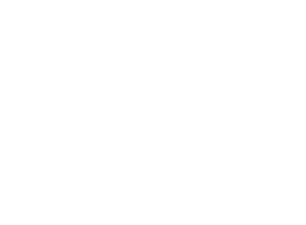In the figure shown below, $ABCDE$ is a regular pentagon and $AG=1$. What is $FG+JH+CD$?

$\textbf{(A) } 3 \qquad\textbf{(B) } 12-4\sqrt5 \qquad\textbf{(C) } \frac{5+2\sqrt5}{3} \qquad\textbf{(D) } 1+\sqrt5 \qquad\textbf{(E) } \frac{11+11\sqrt5}{10}$

AMC 10A, 2014, Problem 9

The two legs of a right triangle, which are altitudes, have lengths $2\sqrt3$ and $6$. How long is the third altitude of the triangle?

$\textbf{(A)}\ 1\qquad\textbf{(B)}\ 2\qquad\textbf{(C)}\ 3\qquad\textbf{(D)}\ 4\qquad\textbf{(E)}\ 5$

AMC 10A, 2014, Problem 12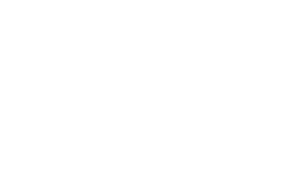A regular hexagon has side length 6. Congruent arcs with radius 3 are drawn with the center at each of the vertices, creating circular sectors as shown. The region inside the hexagon but outside the sectors is shaded as shown. What is the area of the shaded region?

$\textbf{(A)}\ 27\sqrt{3}-9\pi\qquad\textbf{(B)}\ 27\sqrt{3}-6\pi\qquad\textbf{(C)}\ 54\sqrt{3}-18\pi\qquad\textbf{(D)}\ 54\sqrt{3}-12\pi\qquad\textbf{(E)}\ 108\sqrt{3}-9\pi$

AMC 10A, 2014, Problem 13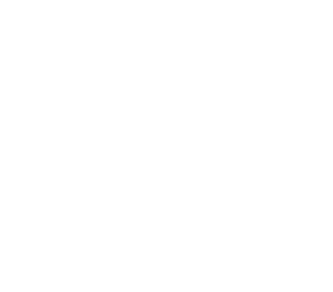Equilateral $\triangle ABC$ has side length $1$, and squares $ABDE$, $BCHI$, $CAFG$ lie outside the triangle. What is the area of hexagon $DEFGHI$?

$\textbf{(A)}\ \frac{12+3\sqrt3}4\qquad\textbf{(B)}\ \frac92\qquad\textbf{(C)}\ 3+\sqrt3\qquad\textbf{(D)}\ \frac{6+3\sqrt3}2\qquad\textbf{(E)}\ 6$

AMC 10A, 2014, Problem 14

The $y$-intercepts, $P$ and $Q$, of two perpendicular lines intersecting at the point $A(6,8)$ have a sum of zero. What is the area of $\triangle APQ$?

$\textbf{(A)}\ 45\qquad\textbf{(B)}\ 48\qquad\textbf{(C)}\ 54\qquad\textbf{(D)}\ 60\qquad\textbf{(E)}\ 72$

AMC 10A, 2014, Problem 16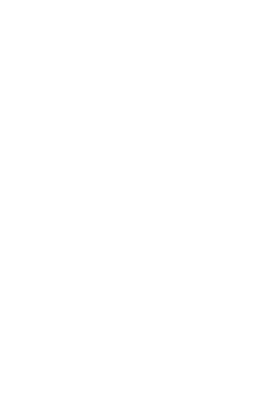In rectangle $ABCD$, $AB=1$, $BC=2$, and points $E$, $F$, and $G$ are midpoints of $\overline{BC}$, $\overline{CD}$, and $\overline{AD}$, respectively. Point $H$ is the midpoint of $\overline{GE}$. What is the area of the shaded region?

$\textbf{(A)}\ \frac1{12}\qquad\textbf{(B)}\ \frac{\sqrt3}{18}\qquad\textbf{(C)}\ \frac{\sqrt2}{12}\qquad\textbf{(D)}\ \frac{\sqrt3}{12}\qquad\textbf{(E)}\ \frac16$

AMC 10A, 2014, Problem 18

A square in the coordinate plane has vertices whose $y$-coordinates are $0$, $1$, $4$, and $5$. What is the area of the square?

$\textbf{(A)}\ 16\qquad\textbf{(B)}\ 17\qquad\textbf{(C)}\ 25\qquad\textbf{(D)}\ 26\qquad\textbf{(E)}\ 27$

AMC 10A, 2014, Problem 19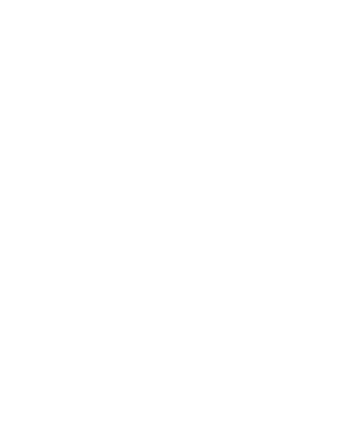Four cubes with edge lengths $1$, $2$, $3$, and $4$ are stacked as shown. What is the length of the portion of $\overline{XY}$ contained in the cube with edge length $3$?

$\textbf{(A)}\ \frac{3\sqrt{33}}5\qquad\textbf{(B)}\ 2\sqrt3\qquad\textbf{(C)}\ \frac{2\sqrt{33}}3\qquad\textbf{(D)}\ 4\qquad\textbf{(E)}\ 3\sqrt2$

AMC 10A, 2014, Problem 22

In rectangle $ABCD$, $AB=20$ and $BC=10$. Let $E$ be a point on $\overline{CD}$ such that $\angle CBE=15^\circ$. What is $AE$?

$\textbf{(A)}\ \frac{20\sqrt3}3\qquad\textbf{(B)}\ 10\sqrt3\qquad\textbf{(C)}\ 18\qquad\textbf{(D)}\ 11\sqrt3\qquad\textbf{(E)}\ 20$

AMC 10A, 2014, Problem 23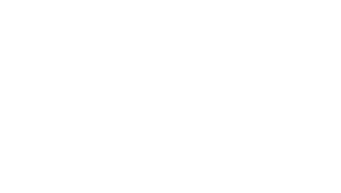A rectangular piece of paper whose length is $\sqrt3$ times the width has area $A$. The paper is divided into three equal sections along the opposite lengths, and then a dotted line is drawn from the first divider to the second divider on the opposite side as shown. The paper is then folded flat along this dotted line to create a new shape with area $B$. What is the ratio $B:A$?

$\textbf{(A)}\ 1:2\qquad\textbf{(B)}\ 3:5\qquad\textbf{(C)}\ 2:3\qquad\textbf{(D)}\ 3:4\qquad\textbf{(E)}\ 4:5$

AMC 10B, 2014, Problem 5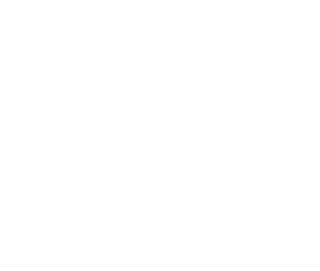Camden constructs a square window using $8$ equal-size panes of glass, as shown. The ratio of the height to width for each pane is $5 : 2$, and the borders around and between the panes are $2$ inches wide. In inches, what is the side length of the square window?

$\textbf{(A)}\ 26\qquad\textbf{(B)}\ 28\qquad\textbf{(C)}\ 30\qquad\textbf{(D)}\ 32\qquad\textbf{(E)}\ 34$

AMC 10B, 2014, Problem 13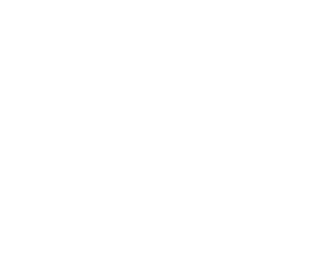Six regular hexagons surround a regular hexagon of side length $1$ as shown. What is the area of $\triangle ABC$?

$\textbf {(A) } 2\sqrt{3} \qquad \textbf {(B) } 3\sqrt{3} \qquad \textbf {(C) } 1+3\sqrt{2} \qquad \textbf {(D) } 2+2\sqrt{3} \qquad \textbf {(E) } 3+2\sqrt{3}$

AMC 10A, 2014, Problem 15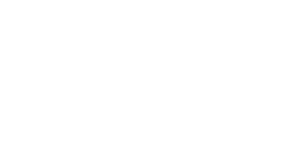In rectangle $ABCD$, $DC=2CB$ and points $E$ and $F$ lie on $\overline{AB}$ so that $\overline{ED}$ and $\overline{FD}$ trisect $\angle ADC$ as shown. What is the ratio of the area of $\triangle DEF$ to the area of rectangle $ABCD$?

$\textbf{(A)}\ \ \frac{\sqrt{3}}{6}\qquad\textbf{(B)}\ \frac{\sqrt{6}}{8}\qquad\textbf{(C)}\ \frac{3\sqrt{3}}{16}\qquad\textbf{(D)}\ \frac{1}{3}\qquad\textbf{(E)}\ \frac{\sqrt{2}}{4}$

AMC 10B, 2014, Problem 19

Two concentric circles have radii $1$ and $2$. Two points on the outer circle are chosen independently and uniformly at random. What is the probability that the chord joining the two points intersects the inner circle?

$\textbf{(A) }\frac{1}{6}\qquad\textbf{(B) }\frac{1}{4}\qquad\textbf{(C) }\frac{2-\sqrt{2}}{2}\qquad\textbf{(D) }\frac{1}{3}\qquad\textbf{(E) }\frac{1}{2}\qquad$

AMC 10B, 2014, Problem 21

Trapezoid $ABCD$ has parallel sides $\overline{AB}$ of length $33$ and $\overline{CD}$ of length $21$. The other two sides are of lengths $10$ and $14$. The angles at $A$ and $B$ are acute. What is the length of the shorter diagonal of $ABCD$?

$\textbf{(A) } 10\sqrt{6} \qquad\textbf{(B) } 25 \qquad\textbf{(C) } 8\sqrt{10} \qquad\textbf{(D) } 18\sqrt{2} \qquad\textbf{(E) } 26$

AMC 10B, 2014, Problem 22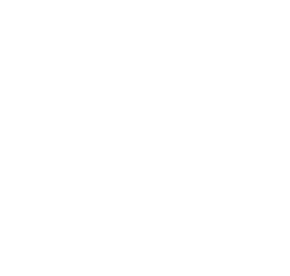Eight semicircles line the inside of a square with side length 2 as shown. What is the radius of the circle tangent to all of these semicircles?

$\text{(A) } \frac{1+\sqrt2}4 \quad \text{(B) } \frac{\sqrt5-1}2 \quad \text{(C) } \frac{\sqrt3+1}4 \quad \text{(D) } \frac{2\sqrt3}5 \quad \text{(E) } \frac{\sqrt5}3$

AMC 10B, 2014, Problem 23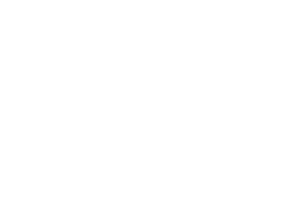A sphere is inscribed in a truncated right circular cone as shown. The volume of the truncated cone is twice that of the sphere. What is the ratio of the radius of the bottom base of the truncated cone to the radius of the top base of the truncated cone?

$\text{(A) } \frac32 \quad \text{(B) } \frac{1+\sqrt5}2 \quad \text{(C) } \sqrt3 \quad \text{(D) } 2 \quad \text{(E) } \frac{3+\sqrt5}2$

AMC 10A, 2013, Problem 13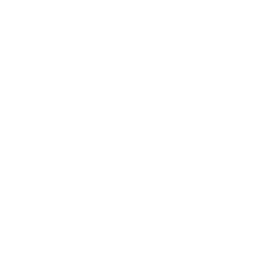Square $ABCD$ has side length $10$. Point $E$ is on $\overline{BC}$, and the area of $\triangle ABE$ is $40$. What is $BE$?

$\textbf{(A)}\ 4\qquad\textbf{(B)}\ 5\qquad\textbf{(C)}\ 6\qquad\textbf{(D)}\ 7\qquad\textbf{(E)}\ 8$

AMC 10A, 2013, Problem 12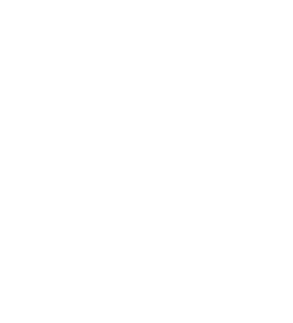n $\triangle ABC$, $AB=AC=28$ and $BC=20$.

Points $D,E,$ and $F$ are on sides $\overline{AB}$, $\overline{BC}$, and $\overline{AC}$, respectively, such that $\overline{DE}$ and $\overline{EF}$ are parallel to $\overline{AC}$ and $\overline{AB}$, respectively. What is the perimeter of parallelogram $ADEF$?

$\textbf{(A) }48\qquad \textbf{(B) }52\qquad \textbf{(C) }56\qquad \textbf{(D) }60\qquad \textbf{(E) }72\qquad$

AMC 10A, 2013, Problem 14

A solid cube of side length $1$ is removed from each corner of a solid cube of side length $3$. How many edges does the remaining solid have?

$\textbf{(A) }36\qquad\textbf{(B) }60\qquad\textbf{(C) }72\qquad\textbf{(D) }84\qquad\textbf{(E) }108\qquad$

AMC 10A, 2013, Problem 15

Two sides of a triangle have lengths $10$ and $15$. The length of the altitude to the third side is the average of the lengths of the altitudes to the two given sides. How long is the third side?

$\textbf{(A)}\ 6 \qquad\textbf{(B)}\ 8 \qquad\textbf{(C)}\ 9 \qquad\textbf{(D)}\ 12 \qquad\textbf{(E)}\ 18$

AMC 10A, 2013, Problem 16

A triangle with vertices $(6, 5)$, $(8, -3)$, and $(9, 1)$ is reflected about the line $x=8$ to create a second triangle. What is the area of the union of the two triangles?

$\textbf{(A)}\ 9 \qquad\textbf{(B)}\ \frac{28}{3} \qquad\textbf{(C)}\ 10 \qquad\textbf{(D)}\ \frac{31}{3} \qquad\textbf{(E)}\ \frac{32}{3}$

AMC 10A, 2013, Problem 18

Let points $A = (0, 0)$, $B = (1, 2)$, $C=(3, 3)$, and $D = (4, 0)$. Quadrilateral $ABCD$ is cut into equal area pieces by a line passing through $A$. This line intersects $\overline{CD}$ at point $\bigg(\frac{p}{q}, \frac{r}{s}\bigg)$, where these fractions are in lowest terms. What is $p+q+r+s$?

$\textbf{(A)}\ 54\qquad\textbf{(B)}\ 58\qquad\textbf{(C)}\ 62\qquad\textbf{(D)}\ 70\qquad\textbf{(E)}\ 75$

AMC 10A, 2013, Problem 20

A unit square is rotated $45^\circ$ about its center. What is the area of the region swept out by the interior of the square?

$\textbf{(A)}\ 1 - \frac{\sqrt2}{2} + \frac{\pi}{4}\qquad\textbf{(B)}\ \frac{1}{2} + \frac{\pi}{4} \qquad\textbf{(C)}\ 2 - \sqrt2 + \frac{\pi}{4}$

$\textbf{(D)}\ \frac{\sqrt2}{2} + \frac{\pi}{4} \qquad\textbf{(E)}\ 1 + \frac{\sqrt2}{4} + \frac{\pi}{8}$

AMC 10A, 2013, Problem 22

Six spheres of radius $1$ are positioned so that their centers are at the vertices of a regular hexagon of side length $2$. The six spheres are internally tangent to a larger sphere whose center is the center of the hexagon. An eighth sphere is externally tangent to the six smaller spheres and internally tangent to the larger sphere. What is the radius of this eighth sphere?

$\textbf{(A)}\ \sqrt2\qquad\textbf{(B)}\ \frac{3}{2}\qquad\textbf{(C)}\ \frac{5}{3}\qquad\textbf{(D)}\ \sqrt3\qquad\textbf{(E)}\ 2$

AMC 10A, 2013, Problem 23

In $\triangle ABC$, $AB = 86$, and $AC=97$. A circle with center $A$ and radius $AB$ intersects $\overline{BC}$ at points $B$ and $X$. Moreover $\overline{BX}$ and $\overline{CX}$ have integer lengths. What is $BC$?

$\textbf{(A)}\ 11\qquad\textbf{(B)}\ 28\qquad\textbf{(C)}\ 33\qquad\textbf{(D)}\ 61\qquad\textbf{(E)}\ 72$

AMC 10A, 2013, Problem 25

All 20 diagonals are drawn in a regular octagon. At how many distinct points in the interior of the octagon (not on the boundary) do two or more diagonals intersect?

$\textbf{(A)}\ 49\qquad\textbf{(B)}\ 65\qquad\textbf{(C)}\ 70\qquad\textbf{(D)}\ 96\qquad\textbf{(E)}\ 128$

AMC 10B, 2013, Problem 2

Mr. Green measures his rectangular garden by walking two of the sides and finding that it is $15$ steps by $20$ steps. Each of Mr. Green's steps is $2$ feet long. Mr. Green expects a half a pound of potatoes per square foot from his garden. How many pounds of potatoes does Mr. Green expect from his garden?

$\textbf{(A)}\ 600 \qquad\textbf{(B)}\ 800 \qquad\textbf{(C)}\ 1000 \qquad\textbf{(D)}\ 1200 \qquad\textbf{(E)}\ 1400$

AMC 10B, 2013, Problem 3

On a particular January day, the high temperature in Lincoln, Nebraska, was $16$ degrees higher than the low temperature, and the average of the high and the low temperatures was $3\,^\circ$. In degrees, what was the low temperature in Lincoln that day?

$\textbf{(A)}\ -13\qquad\textbf{(B)}\ -8\qquad\textbf{(C)}\ -5\qquad\textbf{(D)}\ -3\qquad\textbf{(E)}\ 11$

AMC 10B, 2013, Problem 7

Six points are equally spaced around a circle of radius 1. Three of these points are the vertices of a triangle that is neither equilateral nor isosceles. What is the area of this triangle?

$\textbf{(A)}\ \frac{\sqrt{3}}{3}\qquad\textbf{(B)}\ \frac{\sqrt{3}}{2}\qquad\textbf{(C)}\ 1\qquad\textbf{(D)}\ \sqrt{2}\qquad\textbf{(E)}\ \text{2}$

AMC 10B, 2013, Problem 15

A wire is cut into two pieces, one of length $a$ and the other of length $b$. The piece of length $a$ is bent to form an equilateral triangle, and the piece of length $b$ is bent to form a regular hexagon. The triangle and the hexagon have equal area. What is $\frac{a}{b}$?

$\textbf{(A)}\ 1\qquad\textbf{(B)}\ \frac{\sqrt{6}}{2}\qquad\textbf{(C)}\ \sqrt{3} \qquad\textbf{(D)}\ 2\qquad\textbf{(E)}\ \frac{3\sqrt{2}}{2}$

AMC 10B, 2013, Problem 16

In triangle $ABC$, medians $AD$ and $CE$ intersect at $P$, $PE=1.5$, $PD=2$, and $DE=2.5$. What is the area of $AEDC$?

$\textbf{(A)}13\qquad\textbf{(B)}13.5\qquad\textbf{(C)}14\qquad\textbf{(D)}14.5\qquad\textbf{(E)}15\qquad$

AMC 10B, 2013, Problem 22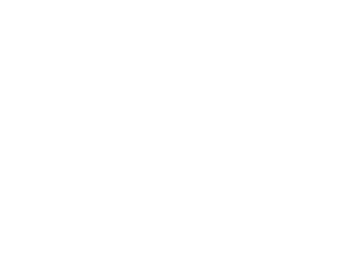The regular octagon $ABCDEFGH$ has its center at $J$. Each of the vertices and the center are to be associated with one of the digits $1$ through $9$, with each digit used once, in such a way that the sums of the numbers on the lines $AJE$, $BJF$, $CJG$, and $DJH$ are all equal. In how many ways can this be done?

$\textbf{(A)}\ 384 \qquad\textbf{(B)}\ 576 \qquad\textbf{(C)}\ 1152 \qquad\textbf{(D)}\ 1680 \qquad\textbf{(E)}\ 3456$

AMC 10B, 2013, Problem 23

In triangle $ABC$, $AB = 13$, $BC = 14$, and $CA = 15$. Distinct points $D$, $E$, and $F$ lie on segments $\overline{BC}$, $\overline{CA}$, and $\overline{DE}$, respectively, such that $\overline{AD} \perp \overline{BC}$, $\overline{DE} \perp \overline{AC}$, and $\overline{AF} \perp \overline{BF}$. The length of segment $\overline{DF}$ can be written as $\frac{m}{n}$, where $m$ and $n$ are relatively prime positive integers. What is $m + n$?

$\textbf{(A)}\ 18\qquad\textbf{(B)}\ 21\qquad\textbf{(C)}\ 24\qquad\textbf{(D)}\ 27\qquad\textbf{(E)}\ 30$

AMC 10A, 2012, Problem 2

A square with side length 8 is cut in half, creating two congruent rectangles. What are the dimensions of one of these rectangles?

$\textbf{(A)}\ 2\ \text{by}\ 4\qquad\textbf{(B)}\ \ 2\ \text{by}\ 6\qquad\textbf{(C)}\ \ 2\ \text{by}\ 8\qquad\textbf{(D)}\ 4\ \text{by}\ 4\qquad\textbf{(E)}\ 4\ \text{by}\ 8$

AMC 10A, 2012, Problem 4

Let $\angle ABC = 24^\circ$ and $\angle ABD = 20^\circ$. What is the smallest possible degree measure for angle CBD?

$\textbf{(A)}\ 0\qquad\textbf{(B)}\ 2\qquad\textbf{(C)}\ 4\qquad\textbf{(D)}\ 6\qquad\textbf{(E)}\ 12$

AMC 10A, 2012, Problem 10

Mary divides a circle into 12 sectors. The central angles of these sectors, measured in degrees, are all integers and they form an arithmetic sequence. What is the degree measure of the smallest possible sector angle?

$\textbf{(A)}\ 5\qquad\textbf{(B)}\ 6\qquad\textbf{(C)}\ 8\qquad\textbf{(D)}\ 10\qquad\textbf{(E)}\ 12$

AMC 10A, 2012, Problem 11

Mary divides a circle into 12 sectors. The central angles of these sectors, measured in degrees, are all integers and they form an arithmetic sequence. What is the degree measure of the smallest possible sector angle?

$\textbf{(A)}\ 5\qquad\textbf{(B)}\ 6\qquad\textbf{(C)}\ 8\qquad\textbf{(D)}\ 10\qquad\textbf{(E)}\ 12$

AMC 10A, 2012, Problem 14

Chubby makes nonstandard checkerboards that have $31$ squares on each side. The checkerboards have a black square in every corner and alternate red and black squares along every row and column. How many black squares are there on such a checkerboard?

$\textbf{(A)}\ 480 \qquad\textbf{(B)}\ 481 \qquad\textbf{(C)}\ 482 \qquad\textbf{(D)}\ 483 \qquad\textbf{(E)}\ 484$

AMC 10A, 2012, Problem 15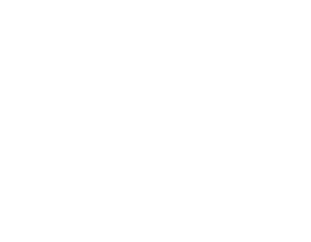Three unit squares and two line segments connecting two pairs of vertices are shown. What is the area of $\triangle ABC$?

$\textbf{(A)}\ \frac16 \qquad\textbf{(B)}\ \frac15 \qquad\textbf{(C)}\ \frac29 \qquad\textbf{(D)}\ \frac13 \qquad\textbf{(E)}\ \frac{\sqrt{2}}{4}$

AMC 10A, 2012, Problem 18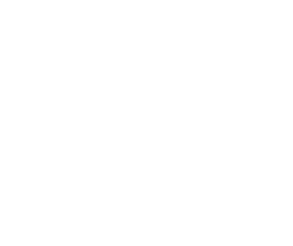The closed curve in the figure is made up of 9 congruent circular arcs each of length $\frac{2\pi}{3}$, where each of the centers of the corresponding circles is among the vertices of a regular hexagon of side 2. What is the area enclosed by the curve?

$\textbf{(A)}\ 2\pi+6\qquad\textbf{(B)}\ 2\pi+4\sqrt{3}\qquad\textbf{(C)}\ 3\pi+4\qquad\textbf{(D)}\ 2\pi+3\sqrt{3}+2\qquad\textbf{(E)}\ \pi+6\sqrt{3}$

AMC 10A, 2012, Problem 21

Let points $A = (0 ,0 ,0)$, $B = (1, 0, 0)$, $C = (0, 2, 0)$, and $D = (0, 0, 3)$. Points $E$, $F$, $G$, and $H$ are midpoints of line segments $\overline{BD},\text{ } \overline{AB}, \text{ } \overline {AC},$ and $\overline{DC}$ respectively. What is the area of $EFGH$?

$\textbf{(A)}\ \sqrt{2}\qquad\textbf{(B)}\ \frac{2\sqrt{5}}{3}\qquad\textbf{(C)}\ \frac{3\sqrt{5}}{4}\qquad\textbf{(D)}\ \sqrt{3}\qquad\textbf{(E)}\ \frac{2\sqrt{7}}{3}$

AMC 10B, 2012, Problem 2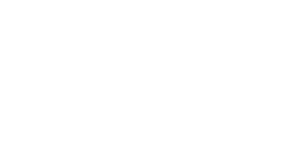A circle of radius 5 is inscribed in a rectangle as shown. The ratio of the length of the rectangle to its width is 2:1. What is the area of the rectangle?

$\textbf{(A)}\ 50\qquad\textbf{(B)}\ 100\qquad\textbf{(C)}\ 125\qquad\textbf{(D)}\ 150\qquad\textbf{(E)}\ 200$

AMC 10B, 2012, Problem 3

The point in the xy-plane with coordinates $(1000, 2012)$ is reflected across the line $y = 2000$. What are the coordinates of the reflected point?

$\textbf{(A)}\ (998,2012)\qquad\textbf{(B)}\ (1000,1988)\qquad\textbf{(C)}\ (1000,2024)\qquad\textbf{(D)}\ (1000,4012)\qquad\textbf{(E)}\ (1012,2012)$

AMC 10B, 2012, Problem 14

Two equilateral triangles are contained in a square whose side length is $2\sqrt 3$. The bases of these triangles are the opposite sides of the square, and their intersection is a rhombus. What is the area of the rhombus?

$\text{(A) } \frac{3}{2} \qquad \text{(B) } \sqrt 3 \qquad \text{(C) } 2\sqrt 2 - 1 \qquad \text{(D) } 8\sqrt 3 - 12 \qquad \text{(E)} \frac{4\sqrt 3}{3}$

AMC 10B, 2012, Problem 16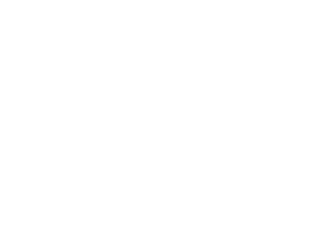Three circles with radius 2 are mutually tangent. What is the total area of the circles and the region bounded by them, as shown in the figure?

$\textbf{(A)}\ 10\pi+4\sqrt{3}\qquad\textbf{(B)}\ 13\pi-\sqrt{3}\qquad\textbf{(C)}\ 12\pi+\sqrt{3}\qquad\textbf{(D)}\ 10\pi+9\qquad\textbf{(E)}\ 13\pi$

AMC 10B, 2012, Problem 17

Jesse cuts a circular paper disk of radius 12 along two radii to form two sectors, the smaller having a central angle of 120 degrees. He makes two circular cones, using each sector to form the lateral surface of a cone. What is the ratio of the volume of the smaller cone to that of the larger?

$\textbf{(A)}\ \frac{1}{8}\qquad\textbf{(B)}\ \frac{1}{4}\qquad\textbf{(C)}\ \frac{\sqrt{10}}{10}\qquad\textbf{(D)}\ \frac{\sqrt{5}}{6}\qquad\textbf{(E)}\ \frac{\sqrt{5}}{5}$

AMC 10B, 2012, Problem 19

In rectangle $ABCD$, $AB=6$, $AD=30$, and $G$ is the midpoint of $\overline{AD}$. Segment $AB$ is extended 2 units beyond $B$ to point $E$, and $F$ is the intersection of $\overline{ED}$ and $\overline{BC}$. What is the area of $BFDG$?

$\textbf{(A)}\ \frac{133}{2}\qquad\textbf{(B)}\ 67\qquad\textbf{(C)}\ \frac{135}{2}\qquad\textbf{(D)}\ 68\qquad\textbf{(E)}\ \frac{137}{2}$

AMC 10B, 2012, Problem 23

A solid tetrahedron is sliced off a wooden unit cube by a plane passing through two nonadjacent vertices on one face and one vertex on the opposite face not adjacent to either of the first two vertices. The tetrahedron is discarded and the remaining portion of the cube is placed on a table with the cut surface face down. What is the height of this object?

$\textbf{(A)}\ \frac{\sqrt{3}}{3}\qquad\textbf{(B)}\ \frac{2\sqrt{2}}{3}\qquad\textbf{(C)}\ 1\qquad\textbf{(D)}\ \frac{2\sqrt{3}}{3}\qquad\textbf{(E)}\ \sqrt{2}$

AMC 10B, 2012, Problem 25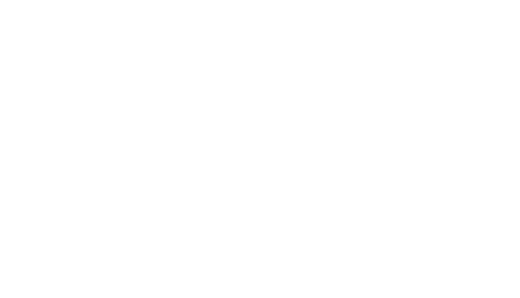A bug travels from $A$ to $B$ along the segments in the hexagonal lattice pictured below. The segments marked with an arrow can be traveled only in the direction of the arrow, and the bug never travels the same segment more than once. How many different paths are there?

$\textbf{(A)}\ 2112\qquad\textbf{(B)}\ 2304\qquad\textbf{(C)}\ 2368\qquad\textbf{(D)}\ 2384\qquad\textbf{(E)}\ 2400$

AMC 10A, 2011, Problem 9

A rectangular region is bounded by the graphs of the equations $y=a, y=-b, x=-c,$ and $x=d$, where $a,b,c,$ and $d$ are all positive numbers. Which of the following represents the area of this region?

$\textbf{(A)}\ ac+ad+bc+bd\qquad\textbf{(B)}\ ac-ad$ $+bc-bd\qquad\textbf{(C)}\ ac+ad$ $-bc-bd \quad\quad\qquad\textbf{(D)}\ -ac-ad$ $+bc+bd\qquad\textbf{(E)}\ ac-ad$ $-bc+bd$

AMC 10A, 2011, Problem 11

Square $EFGH$ has one vertex on each side of square $ABCD$. Point $E$ is on $\overline{AB}$ with $AE=7\cdot EB$. What is the ratio of the area of $EFGH$ to the area of $ABCD$?

$\textbf{(A)}\,\frac{49}{64} \qquad\textbf{(B)}\,\frac{25}{32} \qquad\textbf{(C)}\,\frac78 \qquad\textbf{(D)}\,\frac{5\sqrt{2}}{8} \qquad\textbf{(E)}\,\frac{\sqrt{14}}{4}$

AMC 10A, 2011, Problem 18

Circles $A, B,$ and $C$ each have radius 1. Circles $A$ and $B$ share one point of tangency. Circle $C$ has a point of tangency with the midpoint of $\overline{AB}$. What is the area inside Circle $C$ but outside circle $A$ and circle $B$ ?

$\textbf{(A)}\ 3 - \frac{\pi}{2} \qquad \textbf{(B)}\ \frac{\pi}{2} \qquad \textbf{(C)}\ 2 \qquad \textbf{(D)}\ \frac{3\pi}{4} \qquad \textbf{(E)}\ 1+\frac{\pi}{2}$

AMC 10A, 2011, Problem 22

Each vertex of convex pentagon $ABCDE$ is to be assigned a color. There are $6$ colors to choose from, and the ends of each diagonal must have different colors. How many different colorings are possible?

$\textbf{(A)}\ 2520\qquad\textbf{(B)}\ 2880\qquad\textbf{(C)}\ 3120\qquad\textbf{(D)}\ 3250\qquad\textbf{(E)}\ 3750$

AMC 10A, 2011, Problem 24

Two distinct regular tetrahedra have all their vertices among the vertices of the same unit cube. What is the volume of the region formed by the intersection of the tetrahedra?

$\textbf{(A)}\ \frac{1}{12}\qquad\textbf{(B)}\ \frac{\sqrt{2}}{12}\qquad\textbf{(C)}\ \frac{\sqrt{3}}{12}\qquad\textbf{(D)}\ \frac{1}{6}\qquad\textbf{(E)}\ \frac{\sqrt{2}}{6}$

AMC 10B, 2011, Problem 3

At a store, when a length is reported as $x$ inches that means the length is at least $x - 0.5$ inches and at most $x + 0.5$ inches. Suppose the dimensions of a rectangular tile are reported as $2$ inches by $3$ inches. In square inches, what is the minimum area for the rectangle?

$\textbf{(A)}\ 3.75 \qquad\textbf{(B)}\ 4.5 \qquad\textbf{(C)}\ 5 \qquad\textbf{(D)}\ 6 \qquad\textbf{(E)}\ 8.75$

AMC 10B, 2011, Problem 7

The sum of two angles of a triangle is $6/5$ of a right angle, and one of these two angles is $30^{\circ}$ larger than the other. What is the degree measure of the largest angle in the triangle?

$\textbf{(A)}\ 69 \qquad\textbf{(B)}\ 72 \qquad\textbf{(C)}\ 90 \qquad\textbf{(D)}\ 102 \qquad\textbf{(E)}\ 108$

AMC 10B, 2011, Problem 9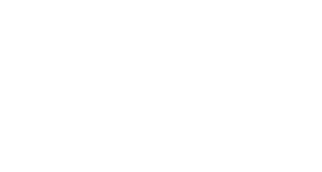The area of $\triangle$$EBD is one third of the area of 3-4-5 \triangle$$ABC$. Segment $DE$ is perpendicular to segment $AB$. What is $BD$?$\textbf{(A)}\ \frac{4}{3} \qquad\textbf{(B)}\ \sqrt{5} \qquad\textbf{(C)}\ \frac{9}{4} \qquad\textbf{(D)}\ \frac{4\sqrt{3}}{3} \qquad\textbf{(E)}\ \frac{5}{2}$

AMC 10B, 2011, Problem 14

A rectangular parking lot has a diagonal of $25$ meters and an area of $168$ square meters. In meters, what is the perimeter of the parking lot?

$\textbf{(A)}\ 52 \qquad\textbf{(B)}\ 58 \qquad\textbf{(C)}\ 62 \qquad\textbf{(D)}\ 68 \qquad\textbf{(E)}\ 70$

AMC 10B, 2011, Problem 16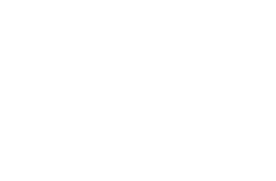A dart board is a regular octagon divided into regions as shown. Suppose that a dart thrown at the board is equally likely to land anywhere on the board. What is probability that the dart lands within the center square?

$\textbf{(A)}\ \frac{\sqrt{2} - 1}{2} \qquad\textbf{(B)}\ \frac{1}{4} \qquad\textbf{(C)}\ \frac{2 - \sqrt{2}}{2} \qquad\textbf{(D)}\ \frac{\sqrt{2}}{4} \qquad\textbf{(E)}\ 2 - \sqrt{2}$

AMC 10B, 2011, Problem 17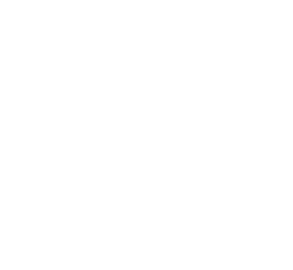In the given circle, the diameter $\overline{EB}$ is parallel to $\overline{DC}$, and $\overline{AB}$ is parallel to $\overline{ED}$. The angles $AEB$ and $ABE$ are in the ratio $4 : 5$. What is the degree measure of angle $BCD$?

$\textbf{(A)}\ 120 \qquad\textbf{(B)}\ 125 \qquad\textbf{(C)}\ 130 \qquad\textbf{(D)}\ 135 \qquad\textbf{(E)}\ 140$

AMC 10B, 2011, Problem 18

Rectangle $ABCD$ has $AB = 6$ and $BC = 3$. Point $M$ is chosen on side $AB$ so that $\angle AMD = \angle CMD$. What is the degree measure of $\angle AMD$?

$\textbf{(A)}\ 15 \qquad\textbf{(B)}\ 30 \qquad\textbf{(C)}\ 45 \qquad\textbf{(D)}\ 60 \qquad\textbf{(E)}\ 75$

AMC 10B, 2011, Problem 20

Rhombus $ABCD$ has side length $2$ and $\angle B = 120$°. Region $R$ consists of all points inside the rhombus that are closer to vertex $B$ than any of the other three vertices. What is the area of $R$?

$\textbf{(A)}\ \frac{\sqrt{3}}{3} \qquad\textbf{(B)}\ \frac{\sqrt{3}}{2} \qquad\textbf{(C)}\ \frac{2\sqrt{3}}{3} \qquad\textbf{(D)}\ 1 + \frac{\sqrt{3}}{3} \qquad\textbf{(E)}\ 2$

AMC 10B, 2011, Problem 22

A pyramid has a square base with sides of length $1$ and has lateral faces that are equilateral triangles. A cube is placed within the pyramid so that one face is on the base of the pyramid and its opposite face has all its edges on the lateral faces of the pyramid. What is the volume of this cube?

$\textbf{(A)}\ 5\sqrt{2} - 7 \qquad\textbf{(B)}\ 7 - 4\sqrt{3} \qquad\textbf{(C)}\ \frac{2\sqrt{2}}{27} \qquad\textbf{(D)}\ \frac{\sqrt{2}}{9} \qquad\textbf{(E)}\ \frac{\sqrt{3}}{9}$

AMC 10B, 2011, Problem 24

A lattice point in an $xy$-coordinate system is any point $(x, y)$ where both $x$ and $y$ are integers. The graph of $y = mx +2$ passes through no lattice point with $0 < x \le 100$ for all $m$ such that $1/2 < m < a$. What is the maximum possible value of $a$?

$\textbf{(A)}\ \frac{51}{101} \qquad\textbf{(B)}\ \frac{50}{99} \qquad\textbf{(C)}\ \frac{51}{100} \qquad\textbf{(D)}\ \frac{52}{101} \qquad\textbf{(E)}\ \frac{13}{25}$

AMC 10B, 2011, Problem 25

Let $T_1$ be a triangle with sides $2011, 2012,$ and $2013$. For $n \ge 1$, if $T_n = \triangle ABC$ and $D, E,$ and $F$ are the points of tangency of the incircle of $\triangle ABC$ to the sides $AB, BC$ and $AC,$ respectively, then $T_{n+1}$ is a triangle with side lengths $AD, BE,$ and $CF,$ if it exists. What is the perimeter of the last triangle in the sequence $( T_n )$?

$\textbf{(A)}\ \frac{1509}{8} \qquad\textbf{(B)}\ \frac{1509}{32} \qquad\textbf{(C)}\ \frac{1509}{64} \qquad\textbf{(D)}\ \frac{1509}{128} \qquad\textbf{(E)}\ \frac{1509}{256}$

AMC 10A, 2010, Problem 2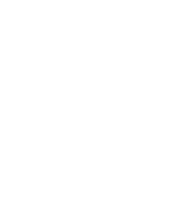Four identical squares and one rectangle are placed together to form one large square as shown. The length of the rectangle is how many times as large as its width?

$\mathrm{(A)}\ \frac{5}{4} \qquad \mathrm{(B)}\ \frac{4}{3} \qquad \mathrm{(C)}\ \frac{3}{2} \qquad \mathrm{(D)}\ 2 \qquad \mathrm{(E)}\ 3$

AMC 10A, 2010, Problem 5

The area of a circle whose circumference is $24\pi$ is $k\pi$. What is the value of $k$?

$\mathrm{(A)}\ 6 \qquad \mathrm{(B)}\ 12 \qquad \mathrm{(C)}\ 24 \qquad \mathrm{(D)}\ 36 \qquad \mathrm{(E)}\ 144$

AMC 10A, 2010, Problem 7

Crystal has a running course marked out for her daily run. She starts this run by heading due north for one mile. She then runs northeast for one mile, then southeast for one mile. The last portion of her run takes her on a straight line back to where she started. How far, in miles, is this last portion of her run?

$\mathrm{(A)}\ 1 \qquad \mathrm{(B)}\ \sqrt{2} \qquad \mathrm{(C)}\ \sqrt{3} \qquad \mathrm{(D)}\ 2 \qquad \mathrm{(E)}\ 2\sqrt{2}$

AMC 10A, 2010, Problem 14

Triangle $ABC$ has $AB=2 \cdot AC$. Let $D$ and $E$ be on $\overline{AB}$ and $\overline{BC}$, respectively, such that $\angle BAE = \angle ACD$. Let $F$ be the intersection of segments $AE$ and $CD$, and suppose that $\triangle CFE$ is equilateral. What is $\angle ACB$?

$\textbf{(A)}\ 60^\circ \qquad \textbf{(B)}\ 75^\circ \qquad \textbf{(C)}\ 90^\circ \qquad \textbf{(D)}\ 105^\circ \qquad \textbf{(E)}\ 120^\circ$

AMC 10A, 2010, Problem 16

Nondegenerate $\triangle ABC$ has integer side lengths, $\overline{BD}$ is an angle bisector, $AD = 3$, and $DC=8$. What is the smallest possible value of the perimeter?

$\textbf{(A)}\ 30 \qquad \textbf{(B)}\ 33 \qquad \textbf{(C)}\ 35 \qquad \textbf{(D)}\ 36 \qquad \textbf{(E)}\ 37$

AMC 10A, 2010, Problem 17

A solid cube has side length $3$ inches. A $2$-inch by $2$-inch square hole is cut into the center of each face. The edges of each cut are parallel to the edges of the cube, and each hole goes all the way through the cube. What is the volume, in cubic inches, of the remaining solid?

$\textbf{(A)}\ 7 \qquad \textbf{(B)}\ 8 \qquad \textbf{(C)}\ 10 \qquad \textbf{(D)}\ 12 \qquad \textbf{(E)}\ 15$

AMC 10A, 2010, Problem 19

Equiangular hexagon $ABCDEF$ has side lengths $AB=CD=EF=1$ and $BC=DE=FA=r$. The area of $\triangle ACE$ is $70\%$ of the area of the hexagon. What is the sum of all possible values of $r$?

$\textbf{(A)}\ \frac{4\sqrt{3}}{3} \qquad \textbf{(B)} \frac{10}{3} \qquad \textbf{(C)}\ 4 \qquad \textbf{(D)}\ \frac{17}{4} \qquad \textbf{(E)}\ 6$

AMC 10A, 2010, Problem 20

A fly trapped inside a cubical box with side length $1$ meter decides to relieve its boredom by visiting each corner of the box. It will begin and end in the same corner and visit each of the other corners exactly once. To get from a corner to any other corner, it will either fly or crawl in a straight line. What is the maximum possible length, in meters, of its path?

$\mathrm{(A)}\ 4+4\sqrt{2} \qquad \mathrm{(B)}\ 2+4\sqrt{2}+2\sqrt{3} \qquad \mathrm{(C)}\ 2+3\sqrt{2}+3\sqrt{3}$
$\mathrm{(D)}\ 4\sqrt{2}+4\sqrt{3} \qquad \mathrm{(E)}\ 3\sqrt{2}+5\sqrt{3}$

AMC 10A, 2010, Problem 22

Eight points are chosen on a circle, and chords are drawn connecting every pair of points. No three chords intersect in a single point inside the circle. How many triangles with all three vertices in the interior of the circle are created?

$\mathrm{(A)}\ 28 \qquad \mathrm{(B)}\ 56 \qquad \mathrm{(C)}\ 70 \qquad \mathrm{(D)}\ 84 \qquad \mathrm{(E)}\ 140$

AMC 10B, 2010, Problem 6

A circle is centered at $O$, $\overline{AB}$ is a diameter and $C$ is a point on the circle with $\angle COB = 50^\circ$. What is the degree measure of $\angle CAB$?

$\textbf{(A)}\ 20 \qquad \textbf{(B)}\ 25 \qquad \textbf{(C)}\ 45 \qquad \textbf{(D)}\ 50 \qquad \textbf{(E)}\ 65$

AMC 10B, 2010, Problem 7

A triangle has side lengths $10$, $10$, and $12$. A rectangle has width $4$ and area equal to the area of the triangle. What is the perimeter of this rectangle?

$\textbf{(A)}\ 16 \qquad \textbf{(B)}\ 24 \qquad \textbf{(C)}\ 28 \qquad \textbf{(D)}\ 32 \qquad \textbf{(E)}\ 36$

AMC 10B, 2010, Problem 16

A square of side length $1$ and a circle of radius $\frac{\sqrt{3}}{3}$ share the same center. What is the area inside the circle, but outside the square?

$\textbf{(A)}\ \frac{\pi}{3}-1 \qquad \textbf{(B)}\ \frac{2\pi}{9}-\frac{\sqrt{3}}{3} \qquad \textbf{(C)}\ \frac{\pi}{18} \qquad \textbf{(D)}\ \frac{1}{4} \qquad \textbf{(E)}\ \frac{2\pi}{9}$

AMC 10B, 2010, Problem 19

A circle with center $O$ has area $156\pi$. Triangle $ABC$ is equilateral, $\overline{BC}$ is a chord on the circle, $OA = 4\sqrt{3}$, and point $O$ is outside $\triangle ABC$. What is the side length of $\triangle ABC$?

$\textbf{(A)}\ 2\sqrt{3} \qquad \textbf{(B)}\ 6 \qquad \textbf{(C)}\ 4\sqrt{3} \qquad \textbf{(D)}\ 12 \qquad \textbf{(E)}\ 18$

AMC 10B, 2010, Problem 20

Two circles lie outside regular hexagon $ABCDEF$. The first is tangent to $\overline{AB}$, and the second is tangent to $\overline{DE}$. Both are tangent to lines $BC$ and $FA$. What is the ratio of the area of the second circle to that of the first circle?

$\textbf{(A)}\ 18 \qquad \textbf{(B)}\ 27 \qquad \textbf{(C)}\ 36 \qquad \textbf{(D)}\ 81 \qquad \textbf{(E)}\ 108$

AMC 10A, 2010, Problem 12

Logan is constructing a scaled model of his town. The city's water tower stands 40 meters high, and the top portion is a sphere that holds 100,000 liters of water. Logan's miniature water tower holds 0.1 liters. How tall, in meters, should Logan make his tower?

$\textbf{(A)}\ 0.04 \qquad \textbf{(B)}\ \frac{0.4}{\pi} \qquad \textbf{(C)}\ 0.4 \qquad \textbf{(D)}\ \frac{4}{\pi} \qquad \textbf{(E)}\ 4$